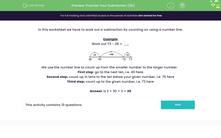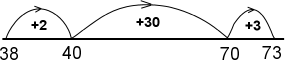### Comprehensive & curriculum aligned

In this worksheet, students work out a subtraction by counting using a number line.Key stage:  KS 2

Curriculum topic:   Number: Addition and Subtraction

Curriculum subtopic:   Solve Add/Subtract Problems to 1000

Popular topics:   Subtraction worksheets

Difficulty level:#### Worksheet Overview

In this worksheet we have to work out a subtraction by counting on using a number line.

Example

Work out 73 - 38 =  __We use the number line to count up from the smaller number to the larger number.

First step: go to the next ten, i.e. 40 here

Second step: count up in tens to the ten below your given number, i.e. 70 here

Third step: count up to the given number, i.e. 73 here

Answer is 2 + 30 + 3 = 35

### What is EdPlace?

We're your National Curriculum aligned online education content provider helping each child succeed in English, maths and science from year 1 to GCSE. With an EdPlace account you’ll be able to track and measure progress, helping each child achieve their best. We build confidence and attainment by personalising each child’s learning at a level that suits them.

Get started